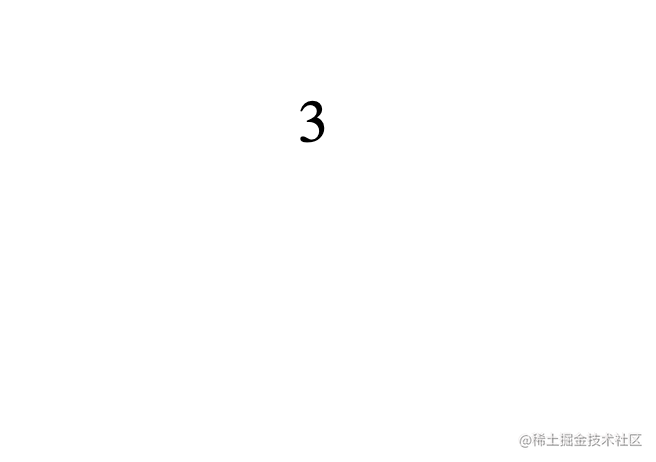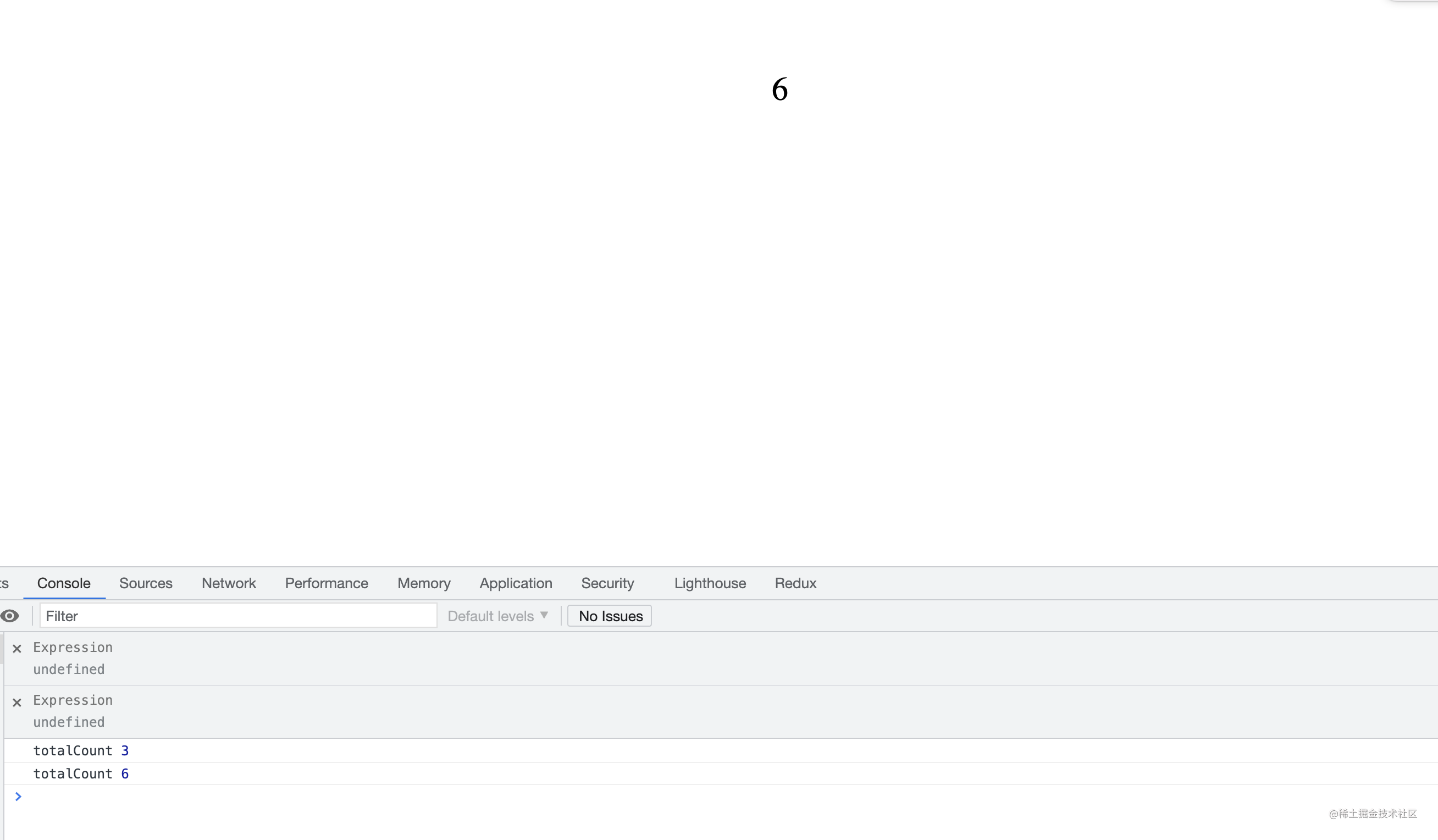# # 手写Vue3 reactive & ref 及对Vue3的响应式原理深度探究

### 什么是响应式数据？🤯🤯🤯

``````let numberOne = 1;
let numberTwo = 2;

document.write(numberOne + numberTwo);``````...

numberOne = 4;

``````let numberA = 1;
let numberB = 2;
let count = numberA + numberB;

console.log("count", count);

numberA = 3;

console.log("count", count);

// 上述的例子里, 如果numberA的值变了以后, count的值也相应变化了, 我们就把numberA称之为响应式数据

• Object.defineProperty
• Proxy
• Object accessor

### ref

``````// 我们的副作用操作
function render() {
document.body.innerText = numberOne.value + numberTwo;
}

``````// 对于ref的代理操作, vue采用的是object accessor的方式
function ref(rawValue) {
return {
get value() {
return rawValue;
},
set value(newValue) {
if (newValue === rawValue) return;
rawValue = newValue;
// 既然这里是一个函数了, 那么我们就可以把我们的副作用操作放在这来
render();
}
}
}

`````` function render() {
document.body.innerText = numberOne.value + numberTwo;
}

function ref(rawValue) {
return {
get value() {
return rawValue;
},
set value(newValue) {
if (newValue === rawValue) return;
rawValue = newValue;
// 既然这里是一个函数了, 那么我们就可以把我们的副作用操作放在这来
render();
}
}
}

let numberOne = ref(1);
let numberTwo = 2;

render(); // 我们先执行一次, 让页面渲染东西, 就和vue的render一样

// 我们修改了页面上的值, 我们看看页面会不会变化
setTimeout(() => {
numberOne.value = 3;
}, 1000)### trace & trigger (依赖收集 / effect触发)

``````// 这个Map是用来干嘛的呢, 一个键名, 对应了依赖这个键的所有副作用函数(用来创建1对多的关系)
// 为啥要用WeakMap, 因为WeakMap的key只能是对象
let depMap = new WeakMap();
let activeEffect = null; // 当前正在运行的effect

// 我为什么要定义这个effect函数来跑副作用函数
// 因为我们知道副作用函数可以有很多, 比如computed, render
// 当我读到某个值的时候, 比如obj.a, 如果我要收集他的依赖
// 我是不是必须在某个副作用函数里, 那比如这个obj.a在副作用函数render中
// 读到的, 是不是代表着我当前运行的effect函数是render
// 所以我用这个effect来跑副作用函数, 是为了帮我记录当前是在哪个副作用环境下
// 所以我们又得出结论: 不在副作用下访问响应式属性 是不用收集依赖的(比如console.log), 听懂掌声
function effect(eff) {
activeEffect = eff;
activeEffect();
activeEffect = null;
}

// trace函数用来帮我们收集键名所对应的effect函数
function trace(target, key) {
if (activeEffect) {
// 我们会干嘛, 会往Map里丢属性和effect
const currentWorkMap = depMap.get(target);

if (!currentWorkMap) {
// 如果这个key没有, 代表这个依赖还是第一次收集吧

// activeEffect是不是一定是当前跑的effect,
// 因为我们必须在某一个effect里才有必要收集依赖
// 而某个effect的执行恰好就是activeEffect的执行吧
depMap.set(target, new Map().set(key, new Set([activeEffect])));
} else {

// 如果不是第一次, 是不是代表这个key对应的value值是一个Map
const matchTargetKey = currentWorkMap.get(key);
if (!matchTargetKey) {
// 代表虽然这个Map有东西, 但是这个key还没有被追踪过
// 为啥要用Set, 因为Set的值不会重复
currentWorkMap.set(key, new Set([activeEffect]));
} else {
// 代表有Set了, 我直接加就行
}
}
}
}

// 我们再来一个trigger方法
// trigger方法是干啥的, 当我们去设置一个响应式数据的值的时候
// 我们是不是希望他对应的所有effect都执行,
// 所以trigger就是用来做这个事儿的
function trigger(target, key) {
const currentMap = depMap.get(target);
if (!currentMap) return; // 如果Map都找不到直接拜拜
const currentWorkEffects = currentMap.get(key);
if (!currentWorkEffects) return;
// 直接将所有的副作用全部执行
currentWorkEffects.forEach(effect => effect());
}

``````function ref(rawValue) {
const proxyObj = {
get value() {
// 取值的时候一定是在某个effect里操作的
// 否则我们就没必要追踪依赖
trace(proxyObj, "value");
return rawValue;
},
set value(newValue) {
if (newValue === rawValue) return;
rawValue = newValue;
trigger(proxyObj, "value");
}
}
}

// 上面就是Set的时候触发, Get的时候追踪了, 非常nice

``````// 副作用操作1
function render() {
document.body.innerText = numberOne.value + numberTwo;
}

// 副作用操作2
let totalCount = 0;
function computedCount() {
totalCount = numberOne.value + numberTwo;
}

...

...

// 我们先跑totalCount的effect
effect(computedCount);

// 然后跑render的effect
effect(render);

console.log("totalCount", totalCount);

// 这个时候我们将numberOne改为4, 我们看一下totalCount的值
numberOne.value = 4;
console.log("totalCount", totalCount);### reactive

``````function reactive(target) {
let handler = {
trace(target, key);
},